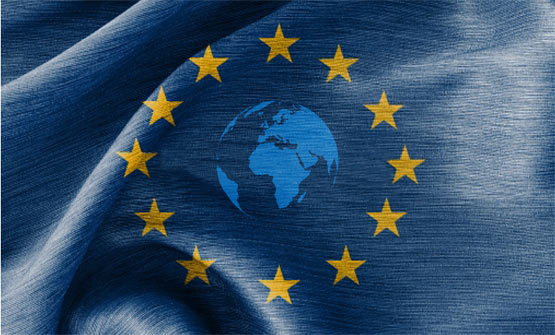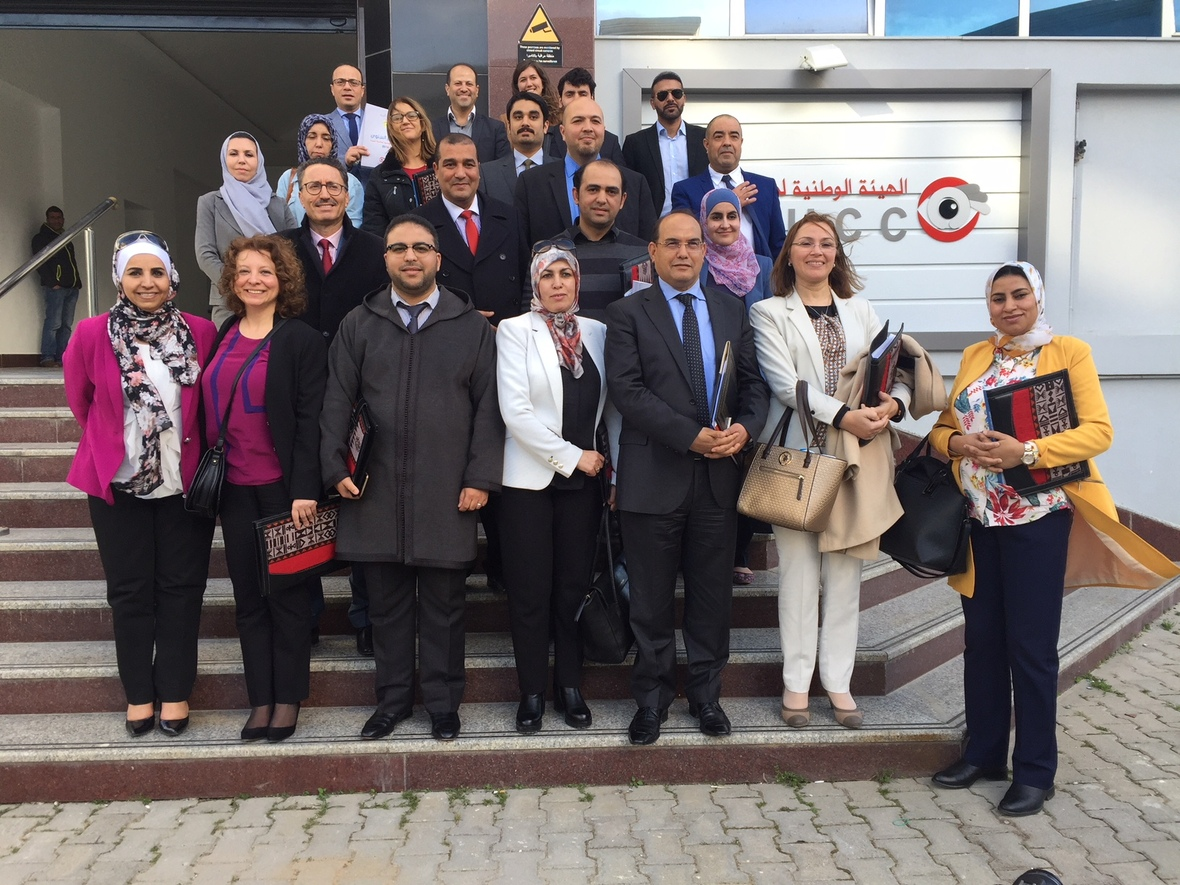Like   Tweet   in

## Asser Today March 2018

 table div table+table+table div table{width:100%;padding:0}table div table+table+table div table img{width:96.23%;padding:0;float:none}table div table+table+table div table td{width:100%;padding:0 1.88% 18px}/* styles */# Asser News

## The International Legality of Economic Activities in Occupied Territories?

The T.M.C. Asser Institute, the Centre for the Law of EU External Relations (CLEER) and The Hague University of Applied Sciences, under the aegis of The Hague Academic Coalition (HAC), invite submissions of abstracts for a workshop on the ‘International Legality of Economic Activities in Occupied Territories’. The workshop will take place at the Asser Institute in The Hague, on 17 October 2018.

## Other Newstable div table+table+table+table+table+table+table+table+table div table,table.module-8{width:50.38%;float:left;padding:0}table div table+table+table+table+table+table+table+table+table div table a{border:0 none;text-decoration:none}table div table+table+table+table+table+table+table+table+table div table img{width:100%!important;border:0 none;text-decoration:none}table div table+table+table+table+table+table+table+table+table div table td{width:100%;padding:0 20px 20px 0}/* styles */
 table div table+table+table+table+table+table+table+table+table+table div table,table.module-9{width:49.62%;float:left;padding:0}table div table+table+table+table+table+table+table+table+table+table div table a{border:0 none;text-decoration:none}table div table+table+table+table+table+table+table+table+table+table div table img{width:100%!important;border:0 none;text-decoration:none}table div table+table+table+table+table+table+table+table+table+table div table td{width:100%;padding:0 20px 20px 0}/* styles *//* styles */

## Selected Publications

▪ Christophe Paulussen and Kate Pitcher, 'Prosecuting (Potential) Foreign Fighters: Legislative and Practical Challenges', ICCT Research Paper, 2018, available at: https://icct.nl/wp-content/uploads/2018/01/ICCT-Paulussen-Pitcher-Prosecuting-Potential-Foreign-Fighters-Legislative-Practical-Challenges-Jan2018.pdf
 ▪ Christophe Paulussen and Kate Pitcher, 'Prosecuting (Potential) Foreign Fighters: Legislative and Practical Challenges', ICCT Research Paper, 2018, available at: https://icct.nl/wp-content/uploads/2018/01/ICCT-Paulussen-Pitcher-Prosecuting-Potential-Foreign-Fighters-Legislative-Practical-Challenges-Jan2018.pdf

 /* styles */ Asser research papers and policy briefs can be downloaded on SSRN.
 table div table+table+table+table+table+table+table+table+table+table+table+table+table+table+table+table div table{width:100%;padding:0}table div table+table+table+table+table+table+table+table+table+table+table+table+table+table+table+table div table img{width:96.23%;padding:0;float:none}table div table+table+table+table+table+table+table+table+table+table+table+table+table+table+table+table div table td{width:100%;padding:0 1.88% 18px}/* styles */# Upcoming Events and Education

The Asser Institute has extensive experience in delivering postgraduate training programmes and in knowledge dissemination in the field of international and European law.

 table div table+table+table+table+table+table+table+table+table+table+table+table+table+table+table+table+table+table div table{width:100%;padding:0}table div table+table+table+table+table+table+table+table+table+table+table+table+table+table+table+table+table+table div table img{width:96.23%;padding:0;float:none}table div table+table+table+table+table+table+table+table+table+table+table+table+table+table+table+table+table+table div table td{width:100%;padding:0 1.88% 18px}/* styles */table div table+table+table+table+table+table+table+table+table+table+table+table+table+table+table+table+table+table+table div table,table.module-18{width:43.77%;float:right;padding:0}table div table+table+table+table+table+table+table+table+table+table+table+table+table+table+table+table+table+table+table div table a{border:0 none;text-decoration:none}table div table+table+table+table+table+table+table+table+table+table+table+table+table+table+table+table+table+table+table div table img{width:100%!important;border:0 none;text-decoration:none}table div table+table+table+table+table+table+table+table+table+table+table+table+table+table+table+table+table+table+table div table td{width:100%;padding:0 0 20px 20px}/* styles */ 12 April Five Years Later: Rana Plaza and the Pursuit of a Responsible Garment Supply Chain The conference brings together key (trans)national policy-makers and civil society members involved in the aftermath of the Rana Plaza collapse. It is designed to serve as a platform for practitioners and academics to reflect on the steps taken to remedy the harm suffered by the victims of the Rana Plaza catastrophe and on the regulatory mechanisms introduced to ensure that it will never reoccur. Our first panel will focus on the avenues available to hold individuals and companies legally accountable for the harm caused by the collapse. The second panel will discuss the transnational (public and private) regulatory responses to the tragedy, addressing health and safety in the Bangladeshi garment industry, and other aspects connected to the regulation of the garment value chain. Finally, the last panel will look at the (soft and hard) regulatory developments at the national level in response to the Rana Plaza collapse. More information and registration
 table div table+table+table+table+table+table+table+table+table+table+table+table+table+table+table+table+table+table+table+table div table,table.module-19{width:71.7%;float:left;padding:0}table div table+table+table+table+table+table+table+table+table+table+table+table+table+table+table+table+table+table+table+table div table a{border:0 none;text-decoration:none}table div table+table+table+table+table+table+table+table+table+table+table+table+table+table+table+table+table+table+table+table div table img{width:100%!important;border:0 none;text-decoration:none}table div table+table+table+table+table+table+table+table+table+table+table+table+table+table+table+table+table+table+table+table div table td{width:100%;padding:0 20px 20px 0}/* styles */ 27 - 31 August Advanced Summer Programme on Countering Terrorism within a Rule of Law Framework This Advanced Summer Programme offers an in-depth look at the challenges that come with adopting and implementing counter-terrorism measures while ensuring respect for human rights, fundamental freedoms and the rule of law. During an intensive week, experts from academia and practice will explore (international) legal aspects of counter-terrorism and provide key insights into current issues and best practices. The programme covers a wide range of topics (including the definition of terrorism in international and domestic law, legal limits to military responses to terrorism, criminal justice responses to terrorism, evidentiary issues and other prosecutorial challenges, administrative and security measures), and comports various types of activities (including lectures, interactive sessions, study visits, group discussions, panel presentations). More information and registration
 table div table+table+table+table+table+table+table+table+table+table+table+table+table+table+table+table+table+table+table+table+table div table,table.module-20{width:71.7%;float:right;padding:0}table div table+table+table+table+table+table+table+table+table+table+table+table+table+table+table+table+table+table+table+table+table div table a{border:0 none;text-decoration:none}table div table+table+table+table+table+table+table+table+table+table+table+table+table+table+table+table+table+table+table+table+table div table img{width:100%!important;border:0 none;text-decoration:none}table div table+table+table+table+table+table+table+table+table+table+table+table+table+table+table+table+table+table+table+table+table div table td{width:100%;padding:0 0 20px 20px}/* styles */ 01 - 05 October Disarmament & Non-Proliferation of WMD Training Programme During this intensive week, participants will be provided with a comprehensive overview of non-proliferation and disarmament efforts regarding Weapons of Mass Destruction. The programme content is delivered by a group of experienced and internationally renowned experts and diplomats in the fields of international law, security and related disciplines. The interactive sessions are complemented with a simulation exercise and field visits to a nuclear reactor and a chemical weapons research laboratory. Four Full scholarships available The Dutch Ministry of Foreign Affairs has generously offered to fund 4 full scholarships. More information and registration
 table div table+table+table+table+table+table+table+table+table+table+table+table+table+table+table+table+table+table+table+table+table+table div table{width:100%;padding:0}table div table+table+table+table+table+table+table+table+table+table+table+table+table+table+table+table+table+table+table+table+table+table div table img{width:96.23%;padding:0;float:none}table div table+table+table+table+table+table+table+table+table+table+table+table+table+table+table+table+table+table+table+table+table+table div table td{width:100%;padding:0 1.88% 18px}/* styles */table.module-22{width:43.21%;padding:0}table div table+table+table+table+table+table+table+table+table+table+table+table+table+table+table+table+table+table+table+table+table+table+table div table{width:43.21%;float:none;margin-left:auto;margin-right:auto;padding:0}table div table+table+table+table+table+table+table+table+table+table+table+table+table+table+table+table+table+table+table+table+table+table+table div table a{border:0 none;text-decoration:none}table div table+table+table+table+table+table+table+table+table+table+table+table+table+table+table+table+table+table+table+table+table+table+table div table img{width:100%!important;border:0 none;text-decoration:none}table div table+table+table+table+table+table+table+table+table+table+table+table+table+table+table+table+table+table+table+table+table+table+table div table td{width:100%;padding:0}/* styles */
 table div table+table+table+table+table+table+table+table+table+table+table+table+table+table+table+table+table+table+table+table+table+table+table+table div table{width:100%;padding:0}table div table+table+table+table+table+table+table+table+table+table+table+table+table+table+table+table+table+table+table+table+table+table+table+table div table img{width:96.23%;padding:0;float:none}table div table+table+table+table+table+table+table+table+table+table+table+table+table+table+table+table+table+table+table+table+table+table+table+table div table td{width:100%;padding:0 1.88% 18px}/* styles */table div table+table+table+table+table+table+table+table+table+table+table+table+table+table+table+table+table+table+table+table+table+table+table+table+table div table,table.module-24{width:32.08%;float:left;padding:0}table div table+table+table+table+table+table+table+table+table+table+table+table+table+table+table+table+table+table+table+table+table+table+table+table+table div table a{border:0 none;text-decoration:none}table div table+table+table+table+table+table+table+table+table+table+table+table+table+table+table+table+table+table+table+table+table+table+table+table+table div table img{width:100%!important;border:0 none;text-decoration:none}table div table+table+table+table+table+table+table+table+table+table+table+table+table+table+table+table+table+table+table+table+table+table+table+table+table div table td{width:100%;padding:0 20px 20px 0}/* styles */ Yearbook of International Sports Arbitration 2016 - Volume 2 Print ISBN 978-94-6265-236-1 EBook/Online ISBN: 978-94-6265-237-8 Distributed for T.M.C. Asser Press by Springer Read this book on SpringerLink
 /* styles */
 table div table+table+table+table+table+table+table+table+table+table+table+table+table+table+table+table+table+table+table+table+table+table+table+table+table+table+table div table{width:100%;padding:0}table div table+table+table+table+table+table+table+table+table+table+table+table+table+table+table+table+table+table+table+table+table+table+table+table+table+table+table div table img{width:96.23%;padding:0;float:none}table div table+table+table+table+table+table+table+table+table+table+table+table+table+table+table+table+table+table+table+table+table+table+table+table+table+table+table div table td{width:100%;padding:0 1.88% 18px}/* styles */# Newsletter of the T.M.C. Asser Instituut

Editor:
Faten Bushehri

The T.M.C. Asser Instituut carries out research on developments in international and European law and its potential for serving the cultivation of trust and respect in the global, regional, national and local societies in which the law operates.

 table div table+table+table+table+table+table+table+table+table+table+table+table+table+table+table+table+table+table+table+table+table+table+table+table+table+table+table+table+table div table{width:100%;padding:0}table div table+table+table+table+table+table+table+table+table+table+table+table+table+table+table+table+table+table+table+table+table+table+table+table+table+table+table+table+table div table img{width:96.23%;padding:0;float:none}table div table+table+table+table+table+table+table+table+table+table+table+table+table+table+table+table+table+table+table+table+table+table+table+table+table+table+table+table+table div table td{width:100%;padding:0 1.88% 18px}/* styles */